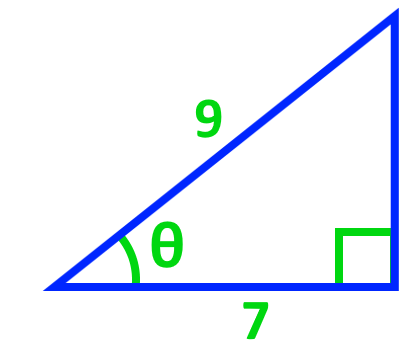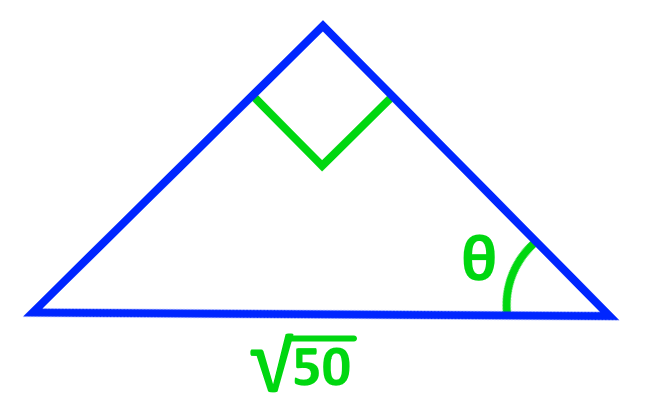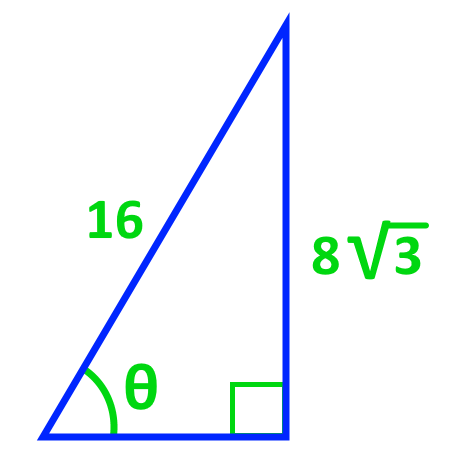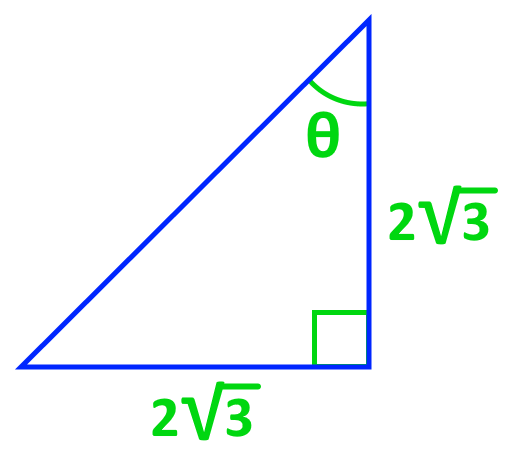# Evaluating inverse trigonometric functions

0/1
##### Introduction
###### Lessons
1. Application of the Cancellation Laws

Introduction to Evaluating Inverse Trigonometric Functions

0/14
##### Examples
###### Lessons
1. Understanding the Use of Inverse Trigonometric Functions

Find the angles for each of the following diagrams.

1.2.2. Find the angle for the following isosceles triangle.1. Determining the Angles in Exact Values by Using Special Triangles

Find the angles for each of the following diagrams in exact value.

1.2.2. Application of the Cancellation Laws

Solve the following inverse trigonometric functions:

1. $\sin (\sin^{-1} 0.5)$
2. $\cos^{-1} (\cos \frac{\pi}{4})$
3. $\sin^{-1} (\sin \frac{3\pi}{4})$
3. Solving Expressions With One Inverse Trigonometry

Solve the following inverse trigonometric functions:

1. $\cos^{-1} \frac{1}{2}$
2. $\sin^{-1} \frac{1}{2}$
4. Evaluating Expressions With a Combination of Inverse and Non-Inverse Trigonometry

Solve the following inverse trigonometric functions:

1. $\sin (\cos^{-1} \frac{\sqrt 3}{2})$
2. $\cos (\sin^{-1} \frac{2}{3})$
3. $\cos (2\tan^{-1} \sqrt 2)$
4. $\cos (\sin^{-1} x)$
5. Special Cases: Evaluating Functions With Numbers Outside of the Restrictions

Solve the following inverse trigonometric functions:

1. $\cos^{-1} (\cos \frac{3\pi}{2})$
2. $\sin^{-1} (\sin \frac{5\pi}{2})$
###### Free to Join!
StudyPug is a learning help platform covering math and science from grade 4 all the way to second year university. Our video tutorials, unlimited practice problems, and step-by-step explanations provide you or your child with all the help you need to master concepts. On top of that, it's fun - with achievements, customizable avatars, and awards to keep you motivated.
• #### Easily See Your ProgressWe track the progress you've made on a topic so you know what you've done. From the course view you can easily see what topics have what and the progress you've made on them. Fill the rings to completely master that section or mouse over the icon to see more details.
• #### Make Use of Our Learning Aids###### Practice Accuracy

See how well your practice sessions are going over time.

Stay on track with our daily recommendations.

• #### Earn Achievements as You LearnMake the most of your time as you use StudyPug to help you achieve your goals. Earn fun little badges the more you watch, practice, and use our service.
• #### Create and Customize Your AvatarPlay with our fun little avatar builder to create and customize your own avatar on StudyPug. Choose your face, eye colour, hair colour and style, and background. Unlock more options the more you use StudyPug.
###### Topic Notes

In this lesson, we will learn:

• Application of the Cancellation Laws
• Solving Expressions With One Inverse Trigonometry
• Evaluating Expressions With a Combination of Inverse and Non-Inverse Trigonometry
• Special Cases: Evaluating Functions With Numbers Outside of the Restrictions

Cancellation Laws:

$\sin^{-1} (\sin x) = x\;$, $-\frac{\pi}{2} \leq x \leq \frac{\pi}{2}$

$\sin (\sin^{-1} x) = x\;$, $-1 \leq x \leq 1$

$\cos^{-1} (\cos x) = x\;$, $0 \leq x \leq \pi$

$\cos (\cos^{-1} x) = x\;$, $-1 \leq x \leq 1$

$\tan^{-1} (\tan x) = x\;$, $-\frac{\pi}{2} \leq x \leq \frac{\pi}{2}$

$\tan (\tan^{-1} x) = x\;$, $-\infty$ < $x$ < $\infty$

Trigonometric Identity:

$\cos 2\theta = \cos^{2} \theta - \sin^{2} \theta$